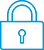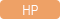cancel
Showing results for
Search instead for
Did you mean:It has been a while since anyone has replied. Simply ask a new question if you would like to start the discussion again.Level 1
8 4 0 0
Message 1 of 5
804
Flag Post

Solved!

# [1 2 3]->a; lu(a); crashes the calculator and reboots it.HP Recommended
HP prime

I accidentally put 1-D array to lu() function as below.

[1 2 3]->a

lu(a)

This crashed the calculator and reboots it again.

When I did the same thing with different calculators, they still showed the answers with crashing.

Tags (1)
4 REPLIES 4Level 8
747 744 121 187
Message 2 of 5
Flag PostHP Recommended

Indeed! I've passed this on to the CAS author for fixing. Thank you!

TW

Although I work for the HP calculator group as a head developer of the HP Prime, the views and opinions I post here are my own.Level 1
8 4 0 0
Message 3 of 5
Flag PostHP Recommended

Another wish for Hp prime lu() improvement is to use the standard algorithm.
It seems that the result of Hp prime lu() is different from those of Matlab and Maxima
while the results from Matlab and Maxima are identical.

a:=[[-2 1 0 0 0]
[1 -2 1 0 0]
[0 1 -2 1 0]
[0 0 1 -2 1]
[0 0 0 1 -2]];
lu(a)

...> So I have writen a hp ppl program as shown below that generates the same answer as Maxima. I wish lu() in hp prime does the same thing as Matlab and Maxima.

#cas
LUP(x):=
BEGIN
LOCAL u,d,m,n,i,j;
LOCAL r,c,p,a,l,k,w;
d:=SIZE(x);
IF DIM(d)==1 THEN
m:=1;
n:=d;
ELSE
m:=d(1);
n:=d(2);
END;
u:=x;
l:=IDENMAT(m);
p:=IDENMAT(m);
c:=1;
k:=0;
FOR r FROM 1 TO m-1 DO
a:=0;
WHILE a==0 AND c=n DO
FOR i FROM r TO m DO
IF u(i,c)?0 THEN
a:=1;
END;
END;
IF a==0 THEN
c:=c+1;
ELSE
k:=k+1;
END;
END;
IF c=n THEN
i:=r;
WHILE u(i,c)==0 AND i=m DO
i:=i+1;
END;
IF i>r THEN
SWAPROW(u,r,i);
SWAPROW(p,r,i);
FOR j FROM 1 TO k-1 DO
a:=l(i,j);
l(i,j):=l(r,j);
l(r,j):=a;
END;
END;
FOR i FROM r+1 TO m DO
a:=u(i,c)/u(r,c);
l[i,k]:=a;
SCALEADD(u,-a,r,i);
END;
END;
END;
return {p,l,expand(simplify(u))};
END;Level 6
305 297 32 76
Message 4 of 5
Flag PostHP Recommended

Prime uses 2 different algorithms.

In Home view it uses LU, which is different from lu in CAS.

CAS can also use LU, just type the command in uppercase.

Besides, the displayal of the result in CAS when using lu is not very clear.

I did some testing and it seems that both methods satisfy the conditions for a LU decomposition.

In both cases all diagonal elements of L are 1.

Tags (1)Level 1
8 4 0 0
Message 5 of 5
Flag PostHP Recommended

Indeed. LU() works like the algorithm of the program LUP that I wrote.

So exact(LU(a)) in CAS mode was equivalent to my program.

I agree that lu() satisfies LU decomposition, but I am using this calculator to self-study a linear algebra textbook where it uses LU instead of lu for Gauss elimination.

† The opinions expressed above are the personal opinions of the authors, not of HP. By using this site, you accept the Terms of Use and Rules of Participation